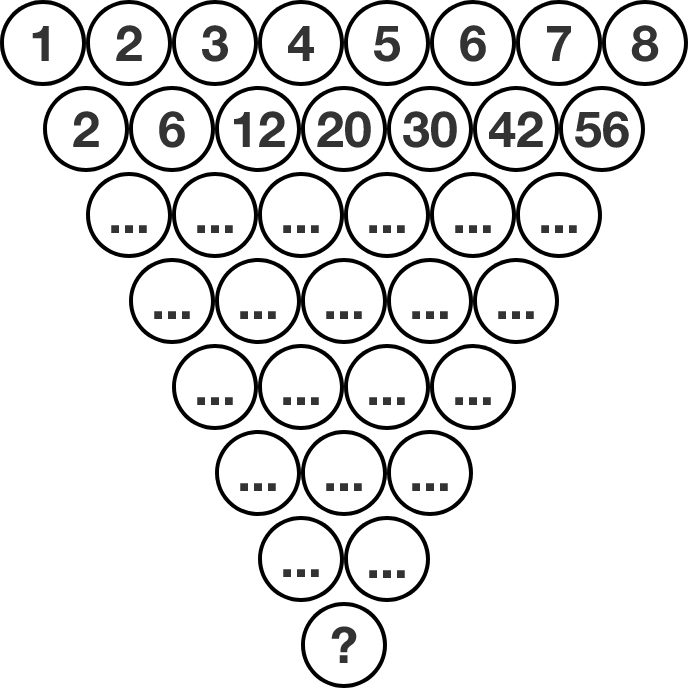# Triangle and numbers

The image shows a triangular formation of circles, where the top row has numbers 1 through 8. The circles in subsequent rows each have the product of the two numbers above it. For example, below the $2$ and $3$ is $2\times 3 = 6.$

How many trailing zeros will the number at the bottom have?×